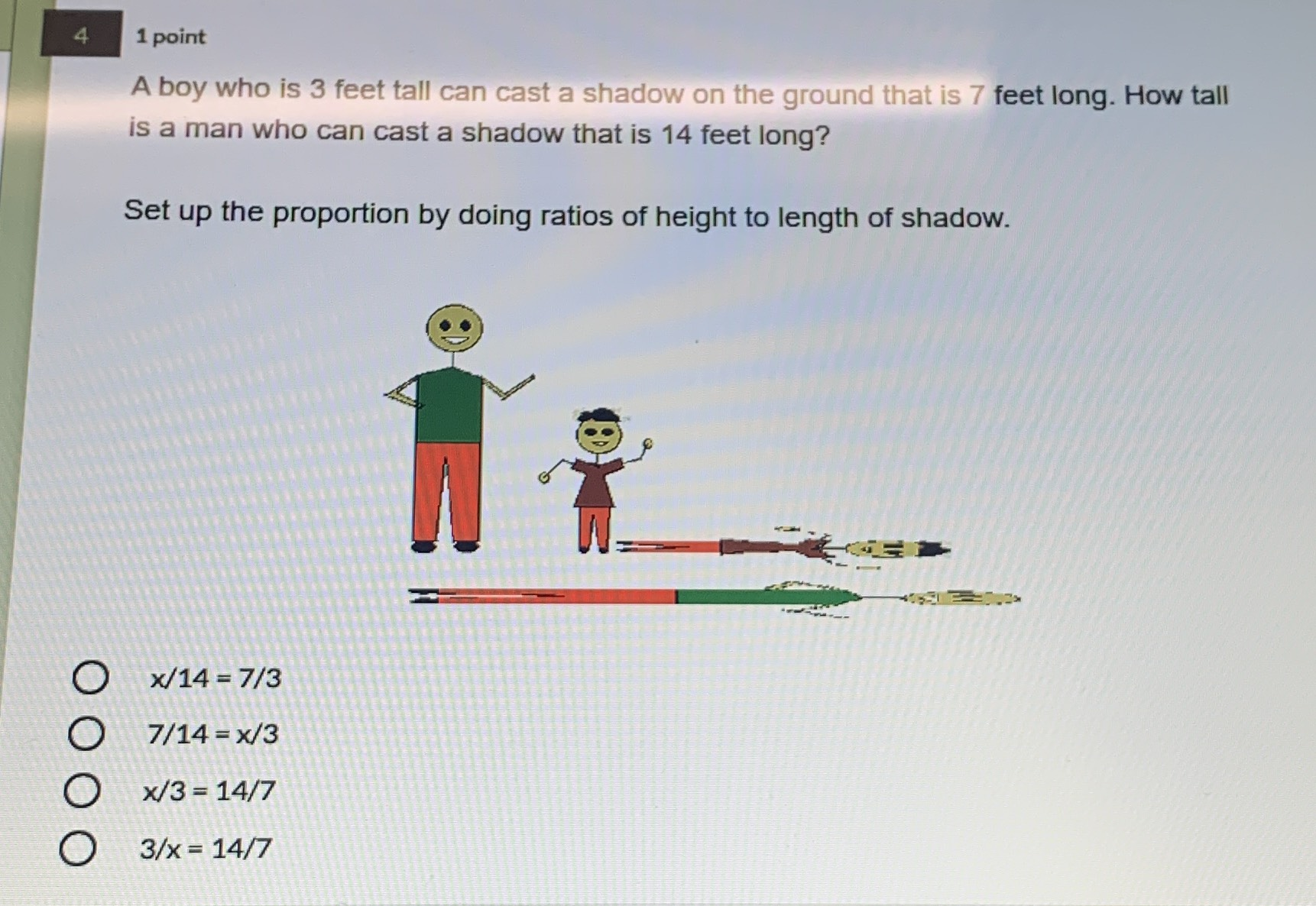### ¿Todavía tienes preguntas de matemáticas?

Pregunte a nuestros tutores expertos
Algebra
PreguntaA boy who is $$3$$ feet tall can cast a shadow on the ground that is $$7$$ feet long. How tall is a man who can cast a shadow that is $$14$$ feet long?

Set up the proportion by doing ratios of height to length of shadow.

$$x / 14 = 7 / 3$$

$$7 / 14 = x / 3$$

$$x / 3 = 14 / 7$$

$$3 / x = 14 / 7$$

$$\frac{x}{3} = \frac{14}{7}$$